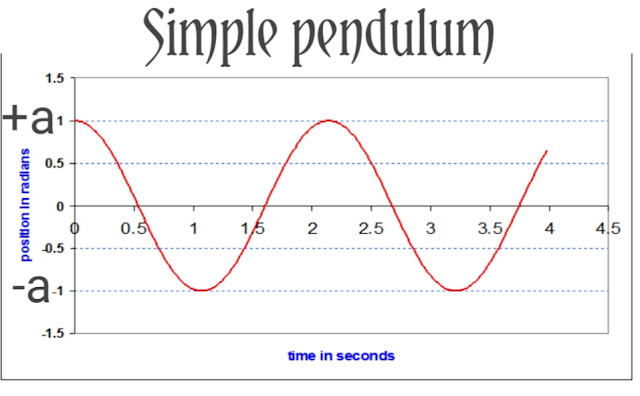# Simple Pendulum

A point connected to a light mass is called a simple pendulum suspended with mass and fixed support.

## Simple pendulum experiment

A simple pendulum consists of a particle of mass ‘m’ usually called bob, suspended from a rigid support with the help of an unstretchable, mass less string. A practical simple pendulum has a small metallic bob suspended from an unstretchable thread and its length l ‘is the distance between the point of suspension and the center of gravity of the bob.

When a simple pendulum is set to oscillate it moves to and fro about it’s mean position between the two extremes A and B with constant amplitude as shown in the figure.Figure 2 showing position the mean and extreme position of a simple pendulum

When we plot  the horizontal displacement and the time on a graph paper the curve obtained would be in the form of a wave.Figure 3 Displacement Vs Timeline of a simple pendulum

Each loop of the graph represents one complete oscillation of the pendulum. When the bob is at its mean position the displacement is zero and at its extremes the displacement is either +a or -a ‘referring to the amplitude of the oscillation.

## Low of semple pendulum

A freely oscillating simple pendulum of a given length always possesses a constant time period and thus it can be used as a time measuring device. Galileo discovered the laws of simple pendulum.

### 1st Law of Simple Pendulum

For small values ​​of amplitude the time period of a simple pendulum of a given length is independent of the amplitude of oscillation.

### 2nd Law of Simple Pendulum

For small values ​​of amplitude the period of a simple pendulum of a given length is independent of its mass, size, density and shape of the bob.

## Verification of lows of simple pendulum

Verification of 1 low

suspend a simple pendulum of length 100 cm from the cork fixed to a retort stand as shown in the figure. Set the simple pendulum to oscillate uniformly about its mean position with a small amplitude, say, 8 cm. Tabulate the time taken by the bob for 20 oscillations in two trials by using a stop watch. Repeat the same procedure by changing the amplitude in two steps say 6 cm and 4 cm. Determine the time period in each case. Determine the average time period of pendulum in each case.

Related post

Thrust

What is force

Units of momentum